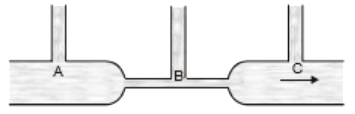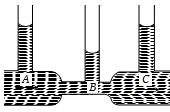#25 | Solved Problems: Set 4:
(Physics) > Mechanical Properties of Fluids
Related Practice Questions :

Bernoulli's theorem based upon

1.  Conservation of momentum

2.  Conservation of energy

3.  Conservation mass

4.  None of these

Complete Question Bank + Test Series
Complete Question Bank

Difficulty Level:

In the figure below is shown the flow of Equid through a horizontal pipe. Three tubes A, B, and C are connected to the pipe. The radii of the tubes, A, B and C at the junction are respectively 2 cm I cm and 2 cm. It can be said that the :1.  Height of the liquid in the tube A is maximum

2.  Height of the liquid in the tubes A and B is the same

3.  Height of the liquid in all the three tubes is the same

4.  Height of the liquid in the tubes A and C is the same

Complete Question Bank + Test Series
Complete Question Bank

Difficulty Level:

Bernoulli's theorem is based on :

1.  conservation of energy

2.  conservation of mass

3.  conservation of momentum

4.  conservation of angular momentum

Complete Question Bank + Test Series
Complete Question Bank

Difficulty Level:

The weight of an aeroplane flying in the air is balanced by

(1) Vertical component of the thrust created by air currents striking the lower surface of the wings

(2) Force due to reaction of gases ejected by the revolving propeller

(3) Upthrust of the air which will be equal to the weight of the air having the same volume as the plane

(4) Force due to the pressure difference between the upper and lower surfaces of the wings created by different air speeds on the surfaces

Complete Question Bank + Test Series
Complete Question Bank

Difficulty Level:

In the following fig. is shown the flow of liquid through a horizontal pipe. Three tubes A, B and C are connected to the pipe. The radii of the tubes A, B and C at the junction are respectively 2 cm, 1 cm and 2 cm. It can be said that the(a) Height of the liquid in the tube A is maximum

(b) Height of the liquid in the tubes A and B is the same

(c) Height of the liquid in all the three tubes is the same

(d) Height of the liquid in the tubes A and C is the same